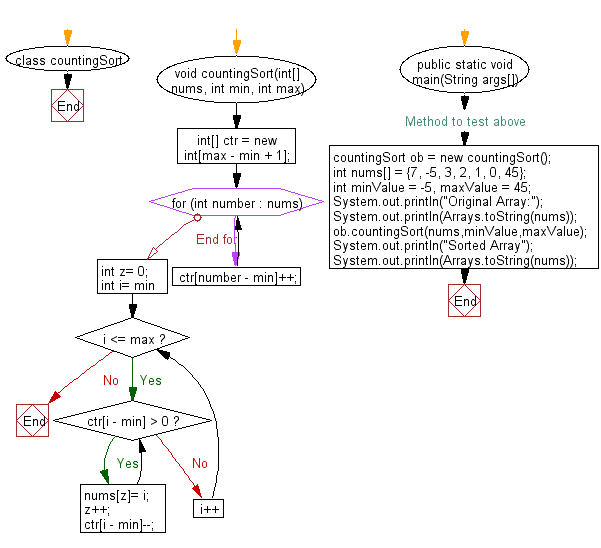﻿ Java exercises: CountingSort Algorithm - w3resource# Java Exercises: CountingSort Algorithm

## Java Sorting Algorithm: Exercise-12 with Solution

Write a Java program to sort an array of given integers using CountingSort Algorithm.

According to Wikipedia "In computer science, counting sort is an algorithm for sorting a collection of objects according to keys that are small integers; that is, it is an integer sorting algorithm. It operates by counting the number of objects that have each distinct key value, and using arithmetic on those counts to determine the positions of each key value in the output sequence. Its running time is linear in the number of items and the difference between the maximum and minimum key values, so it is only suitable for direct use in situations where the variation in keys is not significantly greater than the number of items. However, it is often used as a subroutine in another sorting algorithm, radix sort, that can handle larger keys more efficiently".

The algorithm loops over the items, computing a histogram of the number of times each key occurs within the input collection. It then performs a prefix sum computation (a second loop, over the range of possible keys) to determine, for each key, the starting position in the output array of the items having that key. Finally, it loops over the items again, moving each item into its sorted position in the output array.

Sample Solution:

Java Code:

``````import java.util.Arrays;
class countingSort {
void countingSort(int[] nums, int min, int max){
int[] ctr = new int[max - min + 1];
for(int number : nums){
ctr[number - min]++;
}
int z= 0;
for(int i= min;i <= max;i++){
while(ctr[i - min] > 0){
nums[z]= i;
z++;
ctr[i - min]--;
}
}
}

// Method to test above
public static void main(String args[])
{
countingSort ob = new countingSort();
int nums[] = {7, -5, 3, 2, 1, 0, 45};
int minValue = -5, maxValue = 45;
System.out.println("Original Array:");
System.out.println(Arrays.toString(nums));
ob.countingSort(nums,minValue,maxValue);
System.out.println("Sorted Array");
System.out.println(Arrays.toString(nums));
}
}
```
```

Sample Output:

```Original Array:
[7, -5, 3, 2, 1, 0, 45]
Sorted Array
[-5, 0, 1, 2, 3, 7, 45]
```

Flowchart:Java Code Editor:

What is the difficulty level of this exercise?

﻿

## Java: Tips of the Day

Array vs ArrayLists:

The main difference between these two is that an Array is of fixed size so once you have created an Array you cannot change it but the ArrayList is not of fixed size. You can create instances of ArrayLists without specifying its size. So if you create such instances of an ArrayList without specifying its size Java will create an instance of an ArrayList of default size.

Once an ArrayList is full it re-sizes itself. In fact, an ArrayList is internally supported by an array. So when an ArrayList is resized it will slow down its performance a bit as the contents of the old Array must be copied to a new Array.

At the same time, it's compulsory to specify the size of an Array directly or indirectly while creating it. And also Arrays can store both primitives and objects while ArrayLists only can store objects.

Ref: https://bit.ly/3o8L2KH